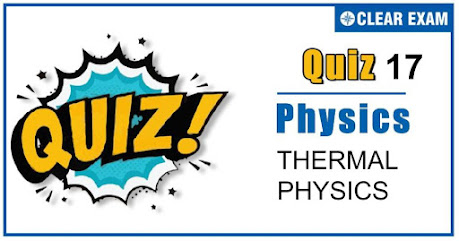## Thermal Physics Quiz-17

As per analysis for previous years, it has been observed that students preparing for NEET find Physics out of all the sections to be complex to handle and the majority of them are not able to comprehend the reason behind it. This problem arises especially because these aspirants appearing for the examination are more inclined to have a keen interest in Biology due to their medical background. Furthermore, sections such as Physics are dominantly based on theories, laws, numerical in comparison to a section of Biology which is more of fact-based, life sciences, and includes substantial explanations. By using the table given below, you easily and directly access to the topics and respective links of MCQs. Moreover, to make learning smooth and efficient, all the questions come with their supportive solutions to make utilization of time even more productive. Students will be covered for all their studies as the topics are available from basics to even the most advanced. .

Q1. A black body radiates at the rate of W watts at a temperature T. If the temperature of the body is reduced to T/3, it will radiate at the rate of (in Watts):
•  W/81
•  W/27
•  W/9
•  W/3
Solution
P=(Q/t)∝T4⇒W/P2 =(T/(T/3))4⇒P2=W/81

Q2. For a small temperature difference between the body and the surroundings the relation between the rate of loss heat R and the temperature of the body is depicted by
Solution
Rate of loss of heat (R)∝ temperature difference ⇒R∝(θ-θ0 )⇒R=k(θ-θ0 )=kθ-kθ0 [k= constant] On comparing it with y=mx+c it is observed that, the graph between R and θ will be straight line with slope =k and intercept =-kθ0

Q3. There are two identical vessels filled with equal amounts of ice. The vessels are of different metals., If the ice melts in the two vessels in 20 and 35 minutes respectively, the ratio of the coefficients of thermal conductivity of the two metals is
•  4 : 7
•  7 : 4
•  16 : 49
•  49 : 16
Solution
Q= (KA(θ12))/l t⇒K1 t1=K2 t2⇒K1/K2 =t2/t1 =35/20=7/4 [As Q,l,A and (θ12) are same]

Q4. The dimensions of thermal resistance are
•  M-1 L-2 T3 K
•  ML2 T-2 K-1
•  ML2 T-3 K
•  ML2 T-2 K-2
Solution
Thermal resistance =l/KA=[L/(MLT-3) K-1×L2 )]=[M-1 L-2 T3 K]

Q5. Five identical rods are joined as shown in figure. Point A and C are maintained at temperature 120℃ and
20℃ respectively. The temperature of junction B will be.
•  100℃
•  80℃
•  70℃
•  0℃
Solution
If thermal resistance of each rod is considered R then, the given combination can be redrawn as follows
(Heat current)AC=(Heat current)AB ((120-20))/2R=((120-θ))/R⇒θ=70℃

Q6. While measuring the thermal conductivity of a liquid, we keep the upper part hot and lower part cool, so that?
•  Convection may be stopped(
•  Heat conduction is easier downwards
•  It is easier and more convenient to do so
Solution
Convection may be stopped.

Q7. A lead bullet of 10 g travelling at 300 m/s strikes against a block of wood and comes to rest. Assuming 50% of heat is absorbed by the bullet, the increase in its temperature is (specific heat of lead =150J/kg,K)
•  100℃
•  125℃
•  150℃
•  200℃
Solution
Since specific heat of lead is given in Joules, hence use W=Q instead of W=JQ. ⇒1/2×(1/2 mv2 )=m.c.∆θ⇒∆θ=v2/4c=(300)2/(4×150)=150℃

Q8. A liquid cools down from 70℃ to 60℃ in 5 minutes. The time taken to cool it from 60℃ to 50℃ will be
•  5 minutes
•  Lesser than 5 minutes
•  Greater than 5 minutes
•  Lesser or greater than 5 minutes depending upon the density of the liquid
Solution
According to Newton’s law of cooling Rate of cooling ∝ mean temperature difference Initially, mean temperature difference =((70+60)/2-θ0 )=(65-θ0) Finally, mean temperature difference =((60+50)/2-θ0 )=(55-θ0) In second case mean temperature difference decreases, so rate of fall of temperature decreases, so it takes more time to cool through the same range

Q9. Two identical bodies have temperatures 277℃ and 67℃ . If the surroundings temperature is 27℃, the ratio of loss of heats of the two bodies during the same interval of time is(approximately)
•  4:1
•  8:1
•  12:1
•  19:1
Solution
T1=277℃=277+273=550 K T2=67℃=67+273=340 K Temperature of surrounding T=27℃=27+273=300 K Ratio of loss of heat=(T14-T4)/(T24-T4 ) =((T1/T)4-1)/((T2/T)4-1)=((550/300)4-1)/((340/300)4-1)=9.5/0.5=19/1

Q10. The absolute zero is the temperature at which:
•  Water freezes
•  All substances exist in solid state
•  Molecular motion ceases
•  None of the above
Solution
At absolute zero (i.e.,0 K)vrms becomes zero## Want to know more

Please fill in the details below:

## Latest NEET Articles\$type=three\$c=3\$author=hide\$comment=hide\$rm=hide\$date=hide\$snippet=hide

Name

ltr
item
BEST NEET COACHING CENTER | BEST IIT JEE COACHING INSTITUTE | BEST NEET & IIT JEE COACHING: Thermal Physics Quiz 17
Thermal Physics Quiz 17
https://1.bp.blogspot.com/-FV1_udBMSgA/X5Ue-XBgJ1I/AAAAAAAABdM/XhljfbvyiB8gMj7WJYTLbRfivuaqKrGeACLcBGAsYHQ/w459-h241/Quiz%2BImage%2BTemplate%2B%252816%2529%2B%25281%2529.jpg
https://1.bp.blogspot.com/-FV1_udBMSgA/X5Ue-XBgJ1I/AAAAAAAABdM/XhljfbvyiB8gMj7WJYTLbRfivuaqKrGeACLcBGAsYHQ/s72-w459-c-h241/Quiz%2BImage%2BTemplate%2B%252816%2529%2B%25281%2529.jpg
BEST NEET COACHING CENTER | BEST IIT JEE COACHING INSTITUTE | BEST NEET & IIT JEE COACHING
https://www.cleariitmedical.com/2020/10/ThermalPhysicsQuiz17.html
https://www.cleariitmedical.com/
https://www.cleariitmedical.com/
https://www.cleariitmedical.com/2020/10/ThermalPhysicsQuiz17.html
true
7783647550433378923
UTF-8

STAY CONNECTED